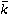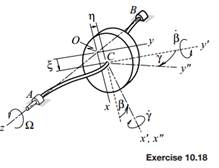### Create an Account

Already have account?

### Forgot Your Password ?

Home / Questions / Whirling is a phenomenon in turbomachinery in which a rotating shaft undergoes displacemen...

# Whirling is a phenomenon in turbomachinery in which a rotating shaft undergoes displacement as a beam with the plane in which the displacement occurs rotating at an angular speed that might differ

Whirling is a phenomenon in turbomachinery in which a rotating shaft undergoes displacement as a beam, with the plane in which the displacement occurs rotating at an angular speed that might differ from the shaft speed. To study this effect, consider the shaft supporting the disk of mass m and radius R to be flexible in bending, rigid in extension and torsion, and massless. Thus the shaft acts like a set of springs that exert forces in opposition to displacement of the disk’s center C, and couples in opposition to the disk’s rotation. The movement of the disk is described by use of several moving coordinate systems. First, there is xyz, which rotates at the shaft speed Ω with its z axis coincident with the centerline between bearings A and B. The origin O of xyz is situated at the location where center C would reside if the shaft were rigid. Coordinate system x y z has center C as its origin, with its y axis always parallel to the y axis. The y axis is the line of nodes for the nutational rotation β of x y z relative to xyz. The third coordinate system, which is xyz in the sketch, is attached to the disk with its origin also at center C. The rotation of this coordinate system relative to x y z is γ about the x axis, which is defined to always coincide with the x axis. (This is an alternative definition of Eulerian angles that also is used in aerospace applications.) The displacement of the center C is ξ+ ηand the angular velocity is Ω+ β˙+ γ˙. In a properly designed system the displacements will be very small compared with the span between bearings and the rotation angles will be sufficiently small to take them to equal their sine. For a shaft of symmetric cross section, each deformation is resisted solely by a corresponding proportional elastic force or moment. Thus the elastic effect of the shaft may be represented by a restoring force −kξ ξ− kηηapplied to the disk at point C and a torque −κγ γ− κβ β, where the small-angle approximation makes it permissible to take= j . Derive the corresponding linearized equations of motion.Jun 16 2020 View more View Less

#### Answer (Solved)Subscribe To Get Solution